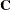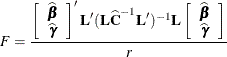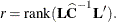Hypothesis Tests for Fixed Effects

Unlike most other SAS/STAT procedures for analyzing general linear models, the HPMIXED procedure does not by default provide F tests for the fixed effects. This is because, for the large mixed model problems that the HPMIXED procedure is designed to address, such tests are often computationally prohibitive to compute. The computation of Type III tests first constructs the Hermite matrix of the mixed model coefficient matrixand then forms thecoefficient matrix to obtain the F value as follows:whereThe coefficient matrixcorresponding to fixed effects with many levels can be very large and dense, making them very difficult to work with. At the same time, Type III tests for effects with many levels are relatively unlikely to be statistically useful.

For this reason, you must use the TEST statement in PROC HPMIXED to specifically ask for Type III tests for any effects for which you want to compute them. An example of this is given in the section Getting Started: HPMIXED Procedure.